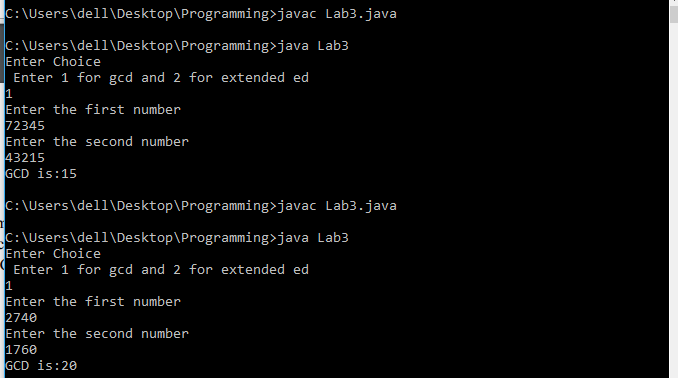# Implement the Pseudo-codes of Euclid’s Algorithm

Let us look into how to implement Euclid’s algorithm and Euclid’s Extended Algorithms using Java Programming Language.

## Euclid’s Algorithm Pseudocode

```int euclid(int a, int b){
if(b==0)
return a;
else
return euclid(b, a%b);
}
```

## Euclid’s Extended Algorithm Pseudocode

```
int exeuclid(int a, int m)
{
int A3 = m;
int A2 = 0, A1 = 1;

if (m == 1)
return 0;

while (a &gt; 1)
{
// q is quotient
int q = a / m;

int t = m;

// m is remainder now, process
// same as Euclid's algo
m = a % m;
a = t;
t = A2;

// Update A1 and A2
A2 = A1 - q * A2;
A1 = t;
}

// Make A1 positive
if (A1 &lt; 0)
A1 += A3;
return A1;
}

```

## Question

Implement the pseudo-codes of Euclid’s algorithm with recursive function and extended Euclid’s algorithm in any programming language you are comfortable with. Your program should take two integers A and B as inputs and give either as GCD (A, B) = d or B-1 = y.

1) Determine the

a) gcd(72345, 43215)

b) gcd(2740, 1760)

2) Find the multiplicative inverse of

a. 550 mod 1769

b. 7465 mod 2464

c. 42828 mod 6407

## Sample Program

```import java.util.Scanner;
class Lab3{
//euclids algorithm
int euclid(int a, int b){
if(b==0)
return a;
else
return euclid(b, a%b);
}
//euclids extended algorithm
int exeuclid(int a, int m)
{
int A3 = m;
int A2 = 0, A1 = 1;
if (m == 1)
return 0;

while (a &gt; 1)
{
// q is quotient
int q = a / m;

int t = m;

// m is remainder now, process
// same as Euclid's algo
m = a % m;
a = t;
t = A2;

// Update A1 and A2
A2 = A1 - q * A2;
A1 = t;
}

// Make A1 positive
if (A1 &lt; 0)
A1 += A3;
return A1;
}
public static void main(String[] args) {
Lab3 ob = new Lab3();
int x, y, choice;

Scanner input = new Scanner(System.in);
System.out.println("Enter Choice \n Enter 1 for gcd and 2 for extended ed");
choice=input.nextInt();
switch(choice)
{
case 1:
int output;
System.out.println("Enter the first number");
x = input.nextInt();
System.out.println("Enter the second number");
y = input.nextInt();
output = ob.euclid(x, y);
System.out.println("GCD is:"+output);
break;
case 2:
System.out.println("To Find Inverse");
int m, b;
System.out.println("Enter m");
m=input.nextInt();
System.out.println("Enter b");
b = input.nextInt();
System.out.println("Inverse is equal to: "+ob.exeuclid(m, b));
break;
default:
System.out.println("Wrong Choice");
}

}
}
```